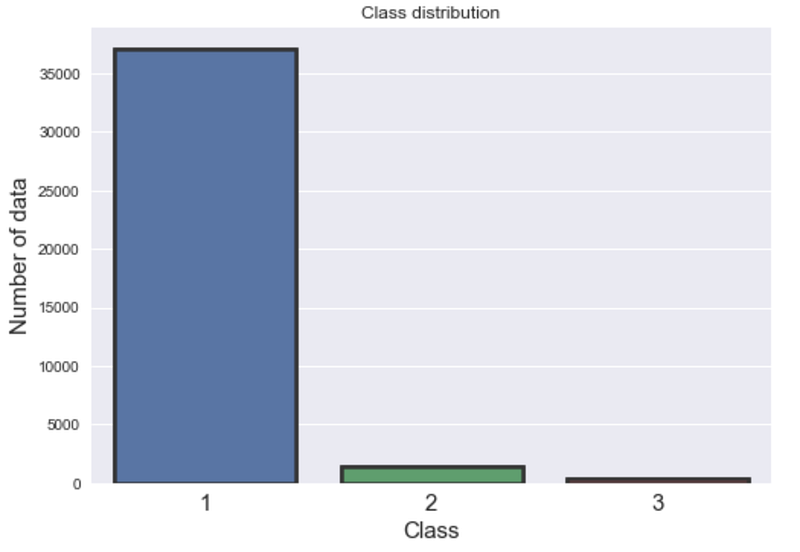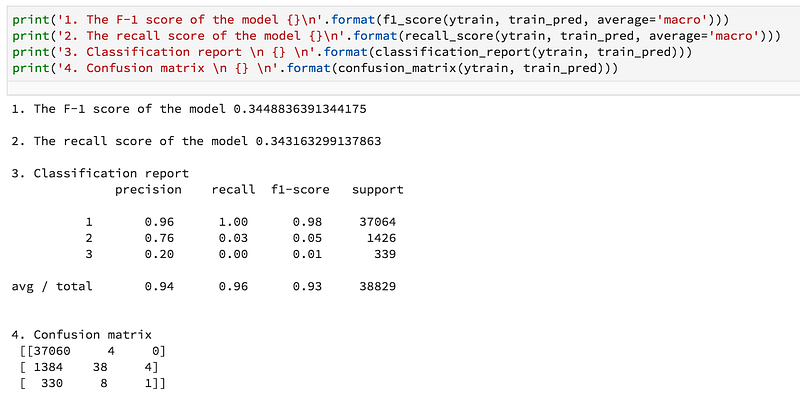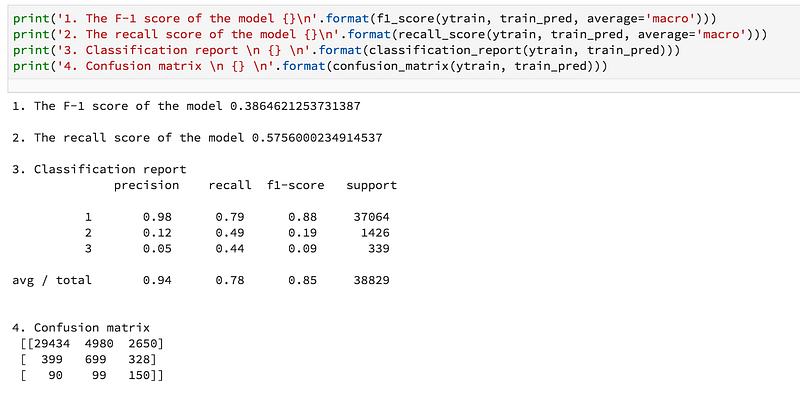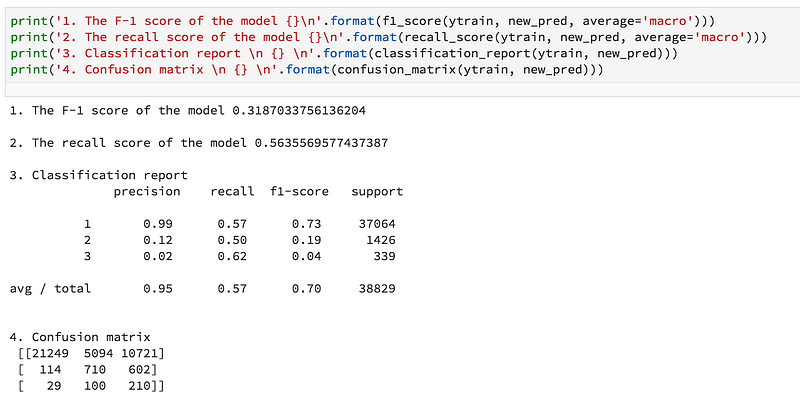# Three techniques to improve machine learning model performance with imbalanced datasets

The primary objective of this project was to handle __data imbalance problem.comments

By Sabber Ahamed, Computational Geophysicist and Machine Learning EnthusiastThis project was part of one my recent job interview skill test for a “Machine learning engineer” position. I had to complete the project in 48 hours which includes writing a 10-page report in latex. The dataset has classes and highly imbalanced. The primary objective of this project was to handle data imbalance issue. In the following subsections, I describe three techniques I used to overcome the data imbalance problem.

First, let’s get started familiarizing with datasets:

Datasets: There are three labels [1, 2, 3] in the training data which makes the problem a multi-class problem. Training datasets have 17 features and 38829 individual data point. Whereas in testing data, there are 16 features without the label and have 16641 data points. The training dataset is very unbalanced. The majority of the data belongs to class-1 (95%) whereas class-2 and class-3 have 3.0% and 0.87% data respectively. Since the datasets do not have any null values and already scaled, I did not do any further processing. Due to some internal reasons, I am not going to share the datasets but the detail results and techniques. The following figure show data imbalance.Figure 1: The graph shows the data imbalance in training dataset. The majority of the data belongs to class-1 (95%) whereas class-2 and class-3 have 3.0% and 0.87% data respectively

Algorithm: After preliminary observation, I decided to use Random forest (RF) algorithm since it outperforms the other algorithms such as support vector machine, Xgboost, LightGBM, etc. RF is a bagging type of ensemble classifier that uses many such single trees to make predictions. There are a couple of reasons for choosing RF in this project:

1. RF is robust to overfitting (thus solving one of the most significant disadvantages of single decision tree).
2. Parameterization remains quite intuitive and straightforward.
3. There are many successful use cases where the random forest algorithm was used in highly unbalanced datasets as we have in this project.
4. I have prior implementation experience of the algorithm.

To find the best parameters, I performed a grid search over specified parameter values using scikit-sklearn implemented GridSearchCV. More details can be found on the Github.

To handle data imbalance issue, I have used the following three techniques :

A. Use Ensemble Cross-Validation (CV): In this project, I used cross-validation to justify the model robustness. The entire datasets were divided into five subsets. In each CV, 4 out of 5 subsets are used for training, and remaining set was used to validate the model. In each CV, the model also predicts (probabilities, not the class) the test data. At the end of the cross-validation, we have five testing prediction probabilities. Finally, I average the prediction probabilities for all class. Training performance of the model was steady and has the almost constant recall and f1 score on each CV. This technique helped me predicting test data very well in one of the Kaggle competitions in which I became top 25th out of 5355 which is top 1%. The following partial code snippets shows the implementation of the Ensemble cross-validation:

```for j, (train_idx, valid_idx) in enumerate(folds):

X_train = X[train_idx]
Y_train = y[train_idx]
X_valid = X[valid_idx]
Y_valid = y[valid_idx]

clf.fit(X_train, Y_train)

valid_pred = clf.predict(X_valid)
recall  = recall_score(Y_valid, valid_pred, average='macro')
f1 = f1_score(Y_valid, valid_pred, average='macro')

recall_scores[i][j] = recall
f1_scores[i][j] = f1

train_pred[valid_idx, i] = valid_pred
test_pred[:, test_col] = clf.predict(T)
test_col += 1

## Probabilities
valid_proba = clf.predict_proba(X_valid)
train_proba[valid_idx, :] = valid_proba
test_proba  += clf.predict_proba(T)

test_proba /= self.n_splits```

B. Set Class Weight/Importance: Cost-sensitive learning is among the many other approaches to make the random forest more suitable for learning from very imbalanced data. The RF has the tendency to be biased on the majority class. Therefore, imposing a costly penalty on the minority class misclassification can be useful. Since this technique is proven the way of improving model performance, I assign a high weight to the minority class (i.e., higher misclassification cost). The class weights are then incorporated into the RF algorithm. I determine a class weight from the ratio between the number of the dataset in class-1 and the number of the dataset in the class. For example, the ratio between the number of datasets in class-1 and class-3 is approximately 110, and the ratio for class-1 and class-2 is about 26. Later, I slightly modify the number for improving the model performance in trail and error basis. The following code snippets show the implementation of the different class weights.

```from sklearn.ensemble import RandomForestClassifier
class_weight = dict({1:1.9, 2:35, 3:180})

rdf = RandomForestClassifier(bootstrap=True,
class_weight=class_weight,
criterion='gini',
max_depth=8, max_features='auto', max_leaf_nodes=None,
min_impurity_decrease=0.0, min_impurity_split=None,
min_samples_leaf=4, min_samples_split=10,
min_weight_fraction_leaf=0.0, n_estimators=300,
oob_score=False,
random_state=random_state,
verbose=0, warm_start=False)```

C. Over-Predict a Label than Under-Predict: This is technique is optional. I have applied this technique since I was asked to implement. It looks to me this method is very effecting to improve minority class performance. In brief, the technique is to penalize the model most if it misclassified class-3, a little less for class-2 and the least for class-1.

To implement the method, I changed the probability threshold for each class. To do so, I set the probability for class-3, class-2 and class-1 in increasing order (i.e, class-3 = 0.25, class-2 = 0.35, class-1 = 0.50), so that the model is forced to over predict class. Detail implementation of this algorithm can be found on this project Github page.

### Final Result:

The following results show that how the above three techniques helped improving the model performance.

1. Result with ensemble cross-validation:2. Result with ensemble cross-validation + class weight:3. Result with ensemble cross-validation + class weight+ over-predict a label:### Conclusion

Since I had minimal experience in implementing the technique, initially over-prediction seems to be tricky to me. However, researching on the method helps me find a way to get around the problem. Due to time constrained I could not focus on fine-tuning and feature engineering of the model. There are many scopes to improve the model further. For example, deleting unnecessary features and adding some extra feature by engineering. I have tried LightGBM and XgBoost as well. But in this short time, I found Random forest outperforms the other algorithms. We might try some other algorithms including a neural network to improve the model. Finally, I would say, from this data challenge I learned how to handle unbalanced data in a well-organized way.

Thank you very much reading. The full code can be found on Github. Let me know if you have any question or this article needs any correction.

Bio: Sabber Ahamed is the Founder of xoolooloo.com. Computational Geophysicist and Machine Learning Enthusiast.

Original. Reposted with permission.

Related:

• 7 Techniques to Handle Imbalanced data
• Learning from Imbalanced Classes
• Dealing with Unbalanced Classes, SVMs, Random Forests, and Decision Trees in Python